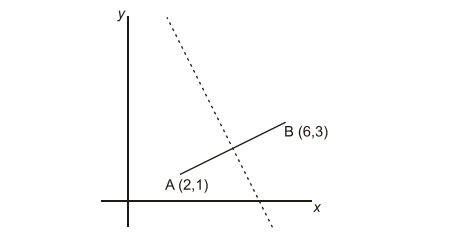# IMAT 2011 Q79 [Joining Graphs]

The graph below shows the line joining A (2,1) and B (6,3), and its perpendicular bisector
(shown dashed: ———-):Which of the following is the equation of the dashed line?

A. y = 2x – 10
B. y = 2x – 6
C. y = 10 – 2x
D. y = 4 – x/2
E. y = 4 – 2x

For these types of questions, we should first find the coordinates of the perpendicular bisector by using the given two points. Also, find the slope perpendicular using the slope point formula. You can also use the midpoint formula to find coordinates.

For point A, let us consider (x1,y1)=(2,1)

For point B, (x2,y2)=(6,3) and slope= m

Now, use formulae to find coordinates. Also, this same equation will give you the slope of the perpendicular bisector by reciprocating and changing the sign.